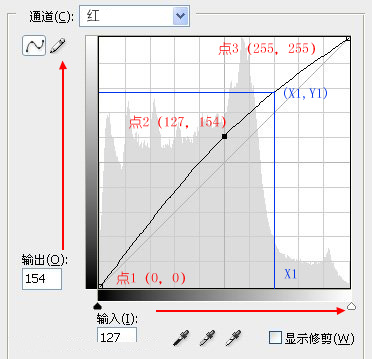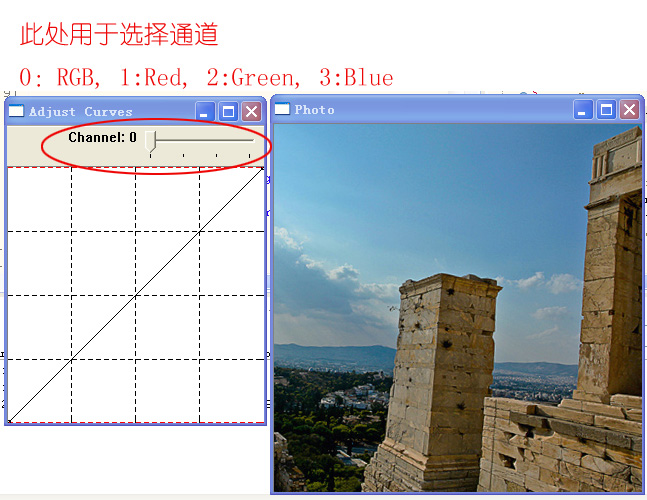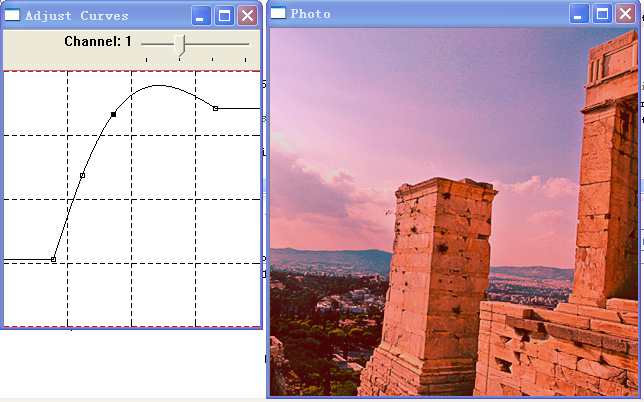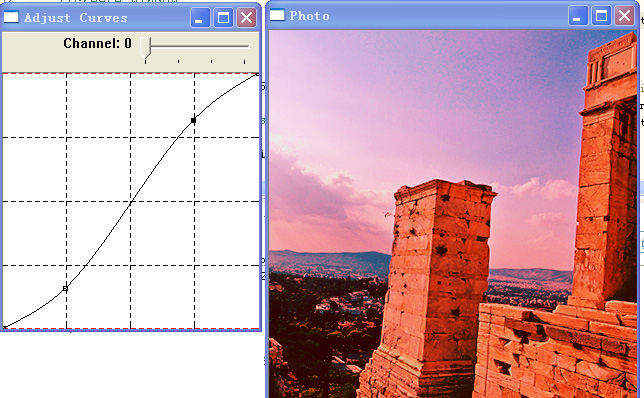（一）曲線原理比如：  畫素點的RGB= (127,  230, 220),  其中紅色值為 X1 = 127,    對應曲線上的值Y1 = 154, 則對該通道曲線調整後 畫素點的RGB= (154,  230, 220)

比如：  畫素點的RGB= (127,  230, 220),  對RGB通道進行整體調整, 則根據該曲線同時對R， G， B三個值進行調整。

R ＝ 127 作為輸入值，  計算曲線上的 對應輸出值  R1

G = 230作為輸入值,   計算曲線上的 對應輸出值  G1

B = 220作為輸入值計算曲線上的 對應輸出值  B1

則新的畫素點的RGB ＝（R1， G1， B1）

（二）曲線的生成

Photoshop使用的曲線是一種SPline 曲線。這種曲線表現力很強，特點是：僅需要定義幾個控制點，就可以定義一條平滑的曲線，且曲線同時通過所有控制點。生成曲線時，只需要給出幾個控制點，呼叫曲線生成函式即可。

SPline的具體數學原理我就不講了，生成函式可以看下面的原始碼Curves.cpp中的spline()函式

（三）曲線調整的OpenCV實現

1， Curves類中定義了四個Curve物件(即四個通道)，分別是RedChannel, GreenChannel, BlueChannel 和 RGBChannel.

2，  Curves類支援用滑鼠生成曲線，使用方法見例程。

2， Curves.cpp中的spline()函式是生成曲線數值的，即輸入一串控制點，通過插值運算，生成一系列的輸出值。

3， 除了用滑鼠生成曲線以外， 也可以用程式程式碼直接生成曲線：

（四）例程

``````/*
* test_Curves.cpp
*
*  Created on: 2016年9月11日
*/

#include <cstdio>
#include <iostream>
#include "opencv2/core.hpp"
#include "opencv2/imgproc.hpp"
#include "opencv2/highgui.hpp"
#include "Curves.hpp"

using namespace std;
using namespace cv;

static string window_name = "Photo";
static Mat src;

static string curves_window = "Adjust Curves";
static Mat curves_mat;
static int channel = 0;
Curves  curves;

static void invalidate()
{
curves.draw(curves_mat);
imshow(curves_window, curves_mat);

Mat dst;
imshow(window_name, dst);

int y, x;
uchar *p;

y = 150; x = 50;
p = dst.ptr<uchar>(y) + x * 3;
cout << "(" << int(p) << ", " << int(p) << ", " << int(p) << ")  ";

y = 150; x = 220;
p = dst.ptr<uchar>(y) + x * 3;
cout << "(" << int(p) << ", " << int(p) << ", " << int(p) << ")  ";

y = 150; x = 400;
p = dst.ptr<uchar>(y) + x * 3;
cout << "(" << int(p) << ", " << int(p) << ", " << int(p) << ")  " << endl;
}

static void callbackAdjustChannel(int , void *)
{
switch (channel) {
case 3:
curves.CurrentChannel = &curves.BlueChannel;
break;
case 2:
curves.CurrentChannel = &curves.GreenChannel;
break;
case 1:
curves.CurrentChannel = &curves.RedChannel;
break;
default:
curves.CurrentChannel = &curves.RGBChannel;
break;
}

invalidate();
}

static void callbackMouseEvent(int mouseEvent, int x, int y, int flags, void* param)
{
switch(mouseEvent) {
case CV_EVENT_LBUTTONDOWN:
curves.mouseDown(x, y);
invalidate();
break;
case CV_EVENT_MOUSEMOVE:
if ( curves.mouseMove(x, y) )
invalidate();
break;
case CV_EVENT_LBUTTONUP:
curves.mouseUp(x, y);
invalidate();
break;
}
return;
}

int main()
{
if ( !src.data ) {
cout << "error read image" << endl;
return -1;
}

//create window
namedWindow(window_name);
imshow(window_name, src);

//create Mat for curves
curves_mat = Mat::ones(256, 256, CV_8UC3);

//create window for curves
namedWindow(curves_window);
setMouseCallback(curves_window, callbackMouseEvent, NULL );

// 範例：用程式程式碼在RedChannel中定義一條曲線
//	curves.RedChannel.clearPoints();

invalidate();

waitKey();

return 0;
}

``````.